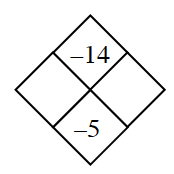### Home > AC > Chapter 7 > Lesson 7.3.1 > Problem7-86

7-86.

Copy and complete each of the Diamond Problems below. The pattern used in the Diamond Problems is shown at right.1.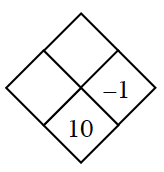$−1\: + \:? = 10$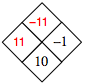1.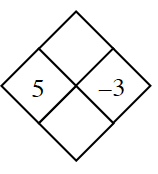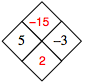1.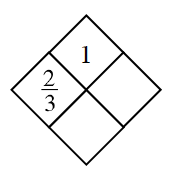$\frac{2}{3}\times y =1$

$\frac{1}{\frac{2}{3}}=x+y$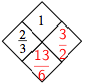1.